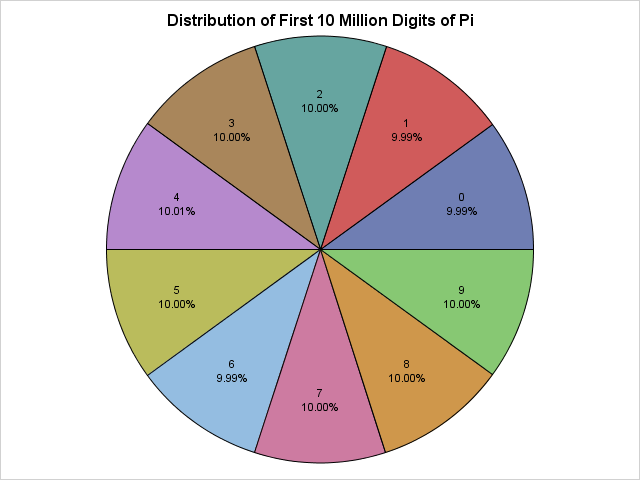Saturday, March 14, 2015, is Pi Day, and this year is a super-special Pi Day! This is your once-in-a-lifetime chance to celebrate the first 10 digits of pi (π) by doing something special on 3/14/15 at 9:26:53. Apologies to my European friends, but Pi Day requires that you represent dates with the month placed first in order to match the sequence 3.141592653....

Last year I celebrated Pi Day by using SAS to explore properties of the continued fraction expansion of pi. This year, I will examine statistical properties of the first 10 million digits of pi. In particular, I will show that the digits of pi exhibit statistical properties that are inherent in a random sequence of integers.

Editor's note (17Mar2015): Ken Kleinman remarked on the similarity of the analysis in this article to his own analysis from 2010. I was reading his blog regularly in 2010, so I should have cited him for the chi-square and Durbin-Watson analyses in this post. My apologies to Ken and Nick Horton for this oversight!

### Reading 10 million digits of pi

I have no desire to type in 10 million digits, so I will use SAS to read a text file at a Princeton University URL. The following statements use the FILENAME statement to point to the URL:

```/* read data over the internet from a URL */ filename rawurl url "http://www.cs.princeton.edu/introcs/data/pi-10million.txt" /* proxy='http://yourproxy.company.com:80' */ ;   data PiDigits; infile rawurl lrecl=10000000; input Digit 1. @@; Position = _n_; Diff = dif(digit); /* compute difference between adjacent digits */ run;   proc print data=PiDigits(obs=9); var Digit; run;```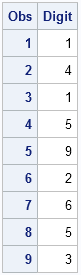The PiDigits data set contains 10 million rows. The call to PROC PRINT displays the first few decimal digits, which are (skipping the 3) 141592653....

For other ways to use SAS to download data from the internet, search Chris Hemedinger's blog, The SAS Dummy for "PROC HTTP" and you will find several examples of how to download data from a URL.

### The distribution of digits of pi

You can run many statistical tests on these numbers. It is conjectured that the digits of pi are randomly uniformly distributed in the sense that the digits 0 through 9 appear equally often, as do pairs of digits, trios of digits, and so forth.

You can call PROC FREQ to compute the frequency distribution of the first 10 million digits of pi and to test whether the digits appear to be uniformly distributed:

```/* Are the digits 0-9 equally distributed? */ proc freq data = PiDigits; tables Digit/ chisq out=DigitFreq; run;```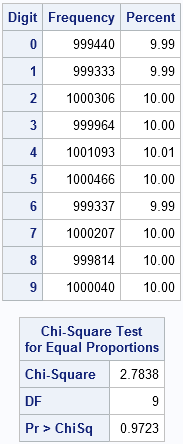The frequency analysis of the first 10 million digits shows that each digit appears about one million times. A chi-square test indicates that the digits appear to be uniformly distributed. If you turn on ODS graphics, PROC FREQ also produces a deviation plot that shows that the deviations from uniformity are tiny.

### A "pi chart" of the distribution of the digits of pi

As an advocate of the #OneLessPie Chart Initiative, I am intellectually opposed to creating pie charts. However, I am going to grit my teeth and make an exception for this super-special Pi Day. You can use the Graph Template Language (GTL) to create a pie chart. Even simpler, Sanjay Matange has written a SAS macro that creates a pie chart with minimal effort. The following DATA step create a percentage variable and then calls Sanjay's macro:

```data DigitFreq; set DigitFreq; Pct = Percent/100; format Pct PERCENT8.2; run;   /* macro from https://blogs.sas.com/content/graphicallyspeaking/2012/08/26/how-about-some-pie/ */ %GTLPieChartMacro(data=DigitFreq, category=Digit, response=Pct, title=Distribution of First 10 Million Digits of Pi, DataSkin=NONE);```

The pie chart appears at the top of this article. It shows that the digits 0 through 9 are equally distributed.

### Any autocorrelation in the sequence?

In the DATA step that read the digits of pi, I calculated the difference between adjacent digits. You can use the SGPLOT procedure to create a histogram that shows the distribution of this quantity:

```proc sgplot data=PiDigits(obs=1000000); vbar Diff; run;```That's a pretty cool triangular distribution! I won't bore you with mathematical details, but this shape arises when you examine the difference between two independent discrete uniform random variables, which suggests that the even digits of pi are independent of the odd digits of pi.

In fact, more is true. You can run a formal test to check for autocorrelation in the sequence of numbers. The Durbin-Watson statistic, which is available in PROC REG and PROC AUTOREG, has a value near 2 if a series of values has no autocorrelation. The following call to PROC AUTOREG requests the Durbin-Watson statistic for first-order through fifth-order autocorrelation for the first one million digits of pi. The results show that there is no detectable autocorrelation through fifth order. To the Durban-Watson test, the digits of pi are indistinguishable from a random sequence:

```proc autoreg data=PiDigits(obs=1000000); model Digit = / dw=5 dwprob; run;```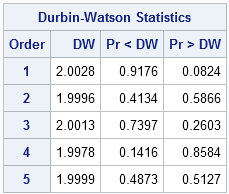### Are the digits of pi random?

Researchers have run dozens of statistical tests for randomness on the digits of pi. They all reach the same conclusion. Statistically speaking, the digits of pi seems to be the realization of a process that spits out digits uniformly at random.

Nevertheless, mathematicians have not yet been able to prove that the digits of pi are random. One of the leading researchers in the quest commented that if they are random then you can find in the sequence (appropriately converted into letters) the "entire works of Shakespeare" or any other message that you can imagine (Bailey and Borwein, 2013). For example, if I assign numeric values to the letters of "Pi Day" (P=16, I=9, D=4, A=1, Y=25), then the sequence "1694125" should appear somewhere in the decimal expansion of pi. I wrote a SAS program to search the decimal expansion of pi for the seven-digit "Pi Day" sequence. Here's what I found:

```proc print noobs data=PiDigits(firstobs=4686485 obs=4686491); var Position Digit; run;```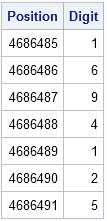There it is! The numeric representation of "Pi Day" appears near the 4.7 millionth decimal place of pi. Other "messages" might not appear in the first 10 million digits, but this one did. Finding Shakespearian sonnets and plays will probably require computing more digits of pi than the current world record.

The digits of pi pass every test for randomness, yet pi is a precise mathematical value that describes the relationship between the circumference of a circle and its diameter. This dichotomy between "very random" and "very structured" is fascinating! Happy Pi Day to everyone!

ShareDistinguished Researcher in Computational Statistics

Rick Wicklin, PhD, is a distinguished researcher in computational statistics at SAS and is a principal developer of SAS/IML software. His areas of expertise include computational statistics, simulation, statistical graphics, and modern methods in statistical data analysis. Rick is author of the books Statistical Programming with SAS/IML Software and Simulating Data with SAS.

1.Rick

Some of your readers will have another chance to celebrate, and this time it will be an international holiday.

data _null_;
call symput('pitime',"21jul2059 00:37:34"dt);
run;
%put &pitime.;

Of course, if you don't want to round, celebrate one second earlier, namely at:
call symput('pitime',"21jul2059 00:37:33"dt);

Art

•Very cool! My new goal is to make that my time of death. I don't know how to manage it, but John Adams, Thomas Jefferson, and James Monroe all died on the 4th of July so I will try.

2.I'm fascinated by the beauty of your autocorrelation analysis... pi - an irrational number with some intriguing properties!

•Ken,
Rick

3.Richard Seibert on

I am curious to see a similar analysis of e, and phi?

Any possibility? Email me direct if you could show me how to do it.

Thanks,

rwsiii

•If you search the internet for
one million digits of "golden ratio"
or
one million digits of e
you will find the data. Have fun!

4.Interesting! There something I do not understand though...If the digits of PI have a uniform distribution based on chi-square 2.78 at 10mill, how come if you calculate chi-square further, at around position 86mill you will get a chi-sqare of 8.9 suggesting it is not uniform.
There is an even smaller chi-square at around 12million (~0.9) so I find it strange that the chi-square would then increase.

•Remember that a statistical test at 0.05 significance will reject a true hypothesis for 5% of random samples. It would not surprise me to find regions in the sequence of pi that test as "nonuniform" for a while. Some of these issues are addressed in "How to lie with a simulation," which coincidentally is also about pi.

5.3.141592... can u have 1000000000000000000000000000000000000000000000000000000000000000000000000000... digits?

•yes

6.Alexei Mironov on

Bad statistic for the First million of digits of Pi !!

7.How can it be that a number so precise and important to math, science, and nature turns out also to be a random number generator? It seems almost self-contradictory.

•Indeed, yet it is true. In fact, almost all real numbers have this property. There are other instances of order within chaos, such as iterations of the logistic map x -> 4*x(1-x), which leads to chaotic dynamics for almost every initial condition, yet also contains infinitely many periodic trajectories. The mathematical universe is strange but beautiful!

•1. If it *weren't* random--if it really did have a pattern--would it then necessarily be rational?
2. Is it possible that pi actually DOES have a pattern that reveals itself, say, after 31.41592653589 trillion digits?

Naive questions, I know. I was only a physics major :)

•1. Pi is definitely irrational, but there are irrational numbers that have patterns. "Irrational" means the decimal expansion is non-terminating and non-repeating. An example that has a pattern is
0.1011011101111011111...
The pattern is groups of ones that repeat 1, 2, 3, 4, ... times. The pattern never repeats, so the number is irrational.
2. Yes, it is possible. It is conjectured that pi is an example of a "normal number," but until the conjecture is proved, no one can be certain.

•Yes, I should have deduced that a pattern of digits can exist without repetition, and thereby still represent an irrational number. But it is irking me that numbers like pi and e might be infinite random sequences of digits, with no underlying structure or pattern...because at least intuitively that seems to imply that the natural phenomena they so beautifully represent are somehow intrinsically random as well. Silly idea, but maybe it's related to the probabilistic nature of quantum physics: If there WERE a pattern (like 0.1011011101111...) then we would KNOW the number even though we couldn't write it all out or represent it as a ratio. But if there is NO pattern or structure, then we can never really pin it down...like an intrinsic uncertainty. We can get closer and closer but never completely represent it. No..no, I'm wrong again, I'm always wrong, because pi and e are easily represented with tidy infinite sums. Aha, so infinite sums can therefore be random number generators?! I see I'm a little too existential, and not quantitative enough, about all this.

•All irrational numbers are random number generators. Even 0.1011011101111011111... is random in a different base.

•I disagree. One of the properties of a (uniform) RNG is that each digit should appear equally often in the output. In your example, after a few iteration, the output is almost always 1. Another property of a good RNG is that it should be impossible to predict the next number from having seen the sequence of previous numbers. In your example, I predict that the next number will be 0.

•Not being random does not imply having a pattern. If you take pi, and replace every '9' by an '8', it isn't random anymore (as it will have no 9's and twice as many 8's as any other digit 0..7), yet it still doesn't have a pattern.

8.when will the frequency of all the digits of pi will be equal till 50 million digits. Or the frequency will always be different

9.Barnaby Finch on

Interesting article! When you say, "Finding Shakespearian sonnets and plays will probably require computing more digits of pi than the current world record," that is a vast understatement. To find an entire Shakespeare play will almost certainly require the computation of more digits, at a rate a trillion times the speed of today's computers, than is possible given the age of the universe.

10.Digits 1-9 show up by the 13th decimal place of pi, but the first 0 does not appear until the 32nd decimal place. For the first 13 places a new digit shows up every digit or to), then 20 places go by before the first 0 appears. That seems very odd if the digits are randomly distributed. But I guess that's the nature of true randomness. Its results are far less uniform that we expect!

•Most humans do not have a good intuition about randomness and probability. The situation you describe is known as the "coupon collector's problem." An easy to visualize situation is rolling a six-sided die and asking how many rolls should you expect to need before you have seen all six faces?

For the digits 0-9, imagine rolling a ten-sided die. How many times do you expect to roll it before all 10 faces have appeared? The answer is 10/10 + 10/9 + 10/8 + ... + 10/1 = 29.3 rolls. So it is not odd at all that you have to look at 32 decimal places in pi until all digits appear!